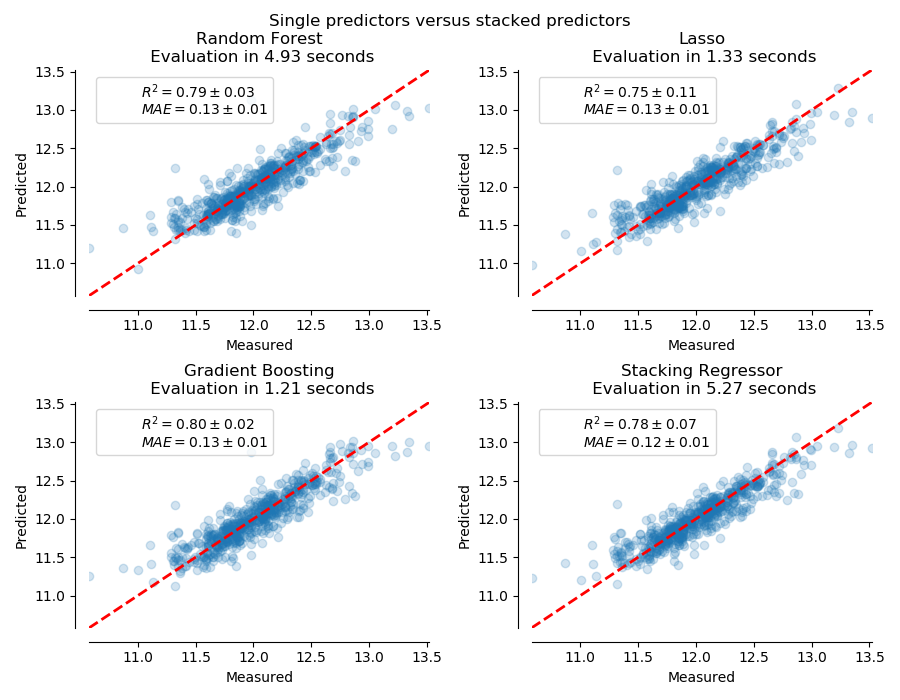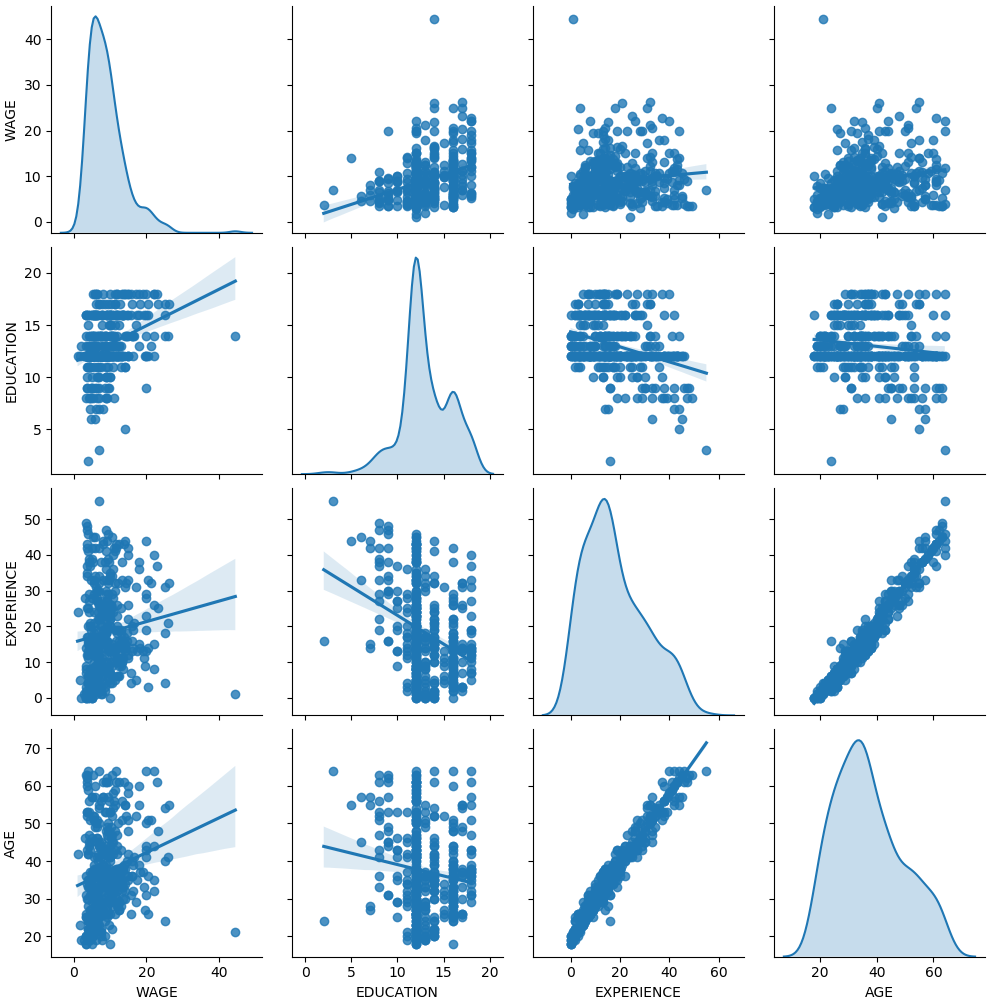# sklearn.model_selection.cross_validate¶

sklearn.model_selection.cross_validate(estimator, X, y=None, *, groups=None, scoring=None, cv=None, n_jobs=None, verbose=0, fit_params=None, pre_dispatch='2*n_jobs', return_train_score=False, return_estimator=False, error_score=nan)

[源码]

estimator estimator object implementing ‘fit’

X array-like of shape (n_samples, n_features)

y array-like of shape (n_samples,) or (n_samples, n_outputs), default=None

groups array-like of shape (n_samples,), default=None

scoring str, callable, list/tuple, or dict, default=None

cv int, cross-validation generator or an iterable, default=None

- None,使用默认的5折交叉验证
- int,用于指定(Stratified)KFold的折数
- CV splitter,
- 可迭代输出切分的(训练集，测试集)作为索引的数组

n_jobs int, default=None

verbose int, default=0

fit_params dict, default=None

pre_dispatch int or str, default=’2*n_jobs’

-None，在这种情况下，将立即创建并派生所有CPU内核。将此用于轻量级和快速运行的CPU内核，以避免由于按需生成CPU内核而造成的延迟
return_train_score bool, default=False

return_estimator bool, default=False

0.20版中的新功能。
error_score ‘raise’ or numeric

0.20版中的新功能。

scores dict of float arrays of shape (n_splits,)

- test_score

- train_score

- fit_time

- score_time

- estimator

sklearn.model_selection.cross_val_score 对单个指标评估进行交叉验证。

sklearn.model_selection.cross_val_predict 从交叉验证的每个切分中获取预测用于诊断。

sklearn.metrics.make_scorer 根据绩效指标或损失函数确定评分器。

>>> from sklearn import datasets, linear_model>>> from sklearn.model_selection import cross_validate>>> from sklearn.metrics import make_scorer>>> from sklearn.metrics import confusion_matrix>>> from sklearn.svm import LinearSVC>>> diabetes = datasets.load_diabetes()>>> X = diabetes.data[:150]>>> y = diabetes.target[:150]>>> lasso = linear_model.Lasso()

>>> cv_results = cross_validate(lasso, X, y, cv=3)>>> sorted(cv_results.keys())['fit_time', 'score_time', 'test_score']>>> cv_results['test_score']array([0.33150734, 0.08022311, 0.03531764])

>>> scores = cross_validate(lasso, X, y, cv=3,...                         scoring=('r2', 'neg_mean_squared_error'),...                         return_train_score=True)>>> print(scores['test_neg_mean_squared_error'])[-3635.5... -3573.3... -6114.7...]>>> print(scores['train_r2'])[0.28010158 0.39088426 0.22784852]

## sklearn.model_selection.cross_validate使用示例¶